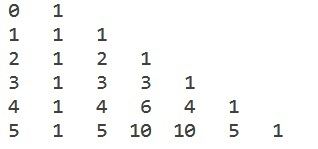# C program for Binomial Coefficients table

Given with a positive integer value let’s say ‘val’ and the task is to print the value of binomial coefficient B(n, k) where, n and k be any value between 0 to val and hence display the result.

## What is Binomial Coefficient

Binomial coefficient (n, k) is the order of choosing ‘k’ results from the given ‘n’ possibilities. The value of binomial coefficient of positive n and k is given by

$$C_k^n=\frac{n!}{(n-k)!k!}$$

where, n >= k

## Example

Input-: B(9,2)
Output-:

$$B_2^9=\frac{9!}{(9-2)!2!}$$

$$\frac{9\times 8\times 7\times 6\times 5\times 4\times 3\times 2\times 1}{6\times 5\times 4\times 3\times 2\times 1)\times 2\times 1}=\frac{362,880}{1440}=252$$

## What is Binomial Coefficient Table

The Binomial Coefficient Table is formed for calculating the multiple values that can be generated between n and k.

## Example

Input-: value = 5
Output-:Approach used in the below program is as follows

• Input the variable ‘val’ from the user for generating the table
• Start the loop from 0 to ‘val’ because the value of binomial coefficient will lie between 0 to ‘val’
• Apply the formula given, if n and k is not 0

B(m, x) = B(m, x - 1) * (m - x + 1) / x

• Print the result

## Algorithm

START
Step 1-> declare function for binomial coefficient table
int bin_table(int val)
Loop For int i = 0 and i <= val and i++
print i
Declare int num = 1
Loop For int j = 0 and j <= i and j++
If (i != 0 && j != 0)
set num = num * (i - j + 1) / j
End
print num
End
print
Step 2-> In main()
Declare int value = 5
call bin_table(value)
STOP

## Example

#include <stdio.h>
// Function for binomial coefficient table
int bin_table(int val) {
for (int i = 0; i <= val; i++) {
printf("%2d", i);
int num = 1;
for (int j = 0; j <= i; j++) {
if (i != 0 && j != 0)
num = num * (i - j + 1) / j;
printf("%4d", num);
}
printf("");
}
}
int main() {
int value = 5;
bin_table(value);
return 0;
}

## Output